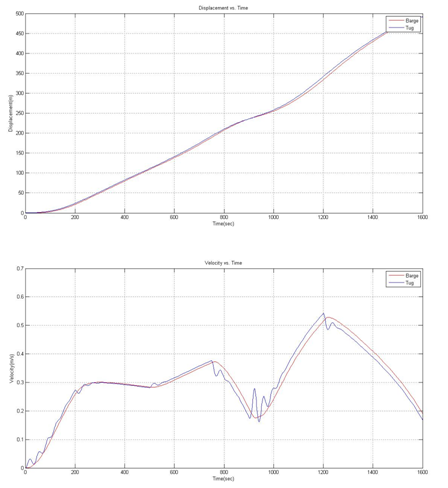## Introduction

General Information about tug and barges### Modeling of the Tug-Barge System

#### Forces Acting On and Inside the System

For constructing a simplified model of the tug-barge system, we consider the forces shown in the following diagram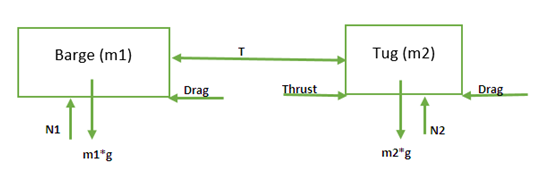Through out the report, the barge will be considered Body 1, and all quantities with sub-script or suffix 1 will be associated with it. Similarly, the index 2 will be used for the tug as a sub-script or suffix.
The next step is to examine the forces acting in vertical and horizontal directions

Vertical Forces
On each of the two bodies, their weight (m1g and m2g respectively) acts downwards while the water surface exerts an upward normal reaction force (N1 and N2 respectively). Under the assumption that there is no vertical motion of the boats, the two forces must cancel each other. So, we conclude that    N1 = m1g and N2 = m2g.

Horizontal Forces
Since the point of interest is the horizontal motion of the boats, it is the horizontal forces which are of chief importance. They are discussed below

Thrust Force:The tug applies a thrust force in the backward direction on the surrounding water through its propellers. The neighboring water applies an equal and opposite force on the tug as per Newton’s Third Law of Motion. This thrust is responsible for the forward motion of the tug, which in turn pulls the barge along with it. The variation in thrust force with time is specified in the problem. The thrust force will be denoted by the term ‘F’ in this report unless explicitly mentioned otherwise.

Drag Force:A body moving in a viscous fluid experiences a force resisting its motion, known as the drag force. The drag force experienced by the body is heavily dependent on the velocity. The dependence varies depending upon the exact geometry, turbulence and other intricacies. The most common assumptions are that the drag force has a linear or quadratic dependence on velocity of the body. In this problem, quadratic dependence has been explicitly specified. In this report, the term ‘f’ will be used exclusively to denote velocity dependent drag force, along with sub-script/suffix 1 or 2 to denote the body on which it acts.

Tension in the tow cable:The tow cable will experience a tension force when the separation between its ends exceeds its natural length (denoted by ‘L’) due to the relative motion of the tug and the barge. The magnitude of the force will be the product of the stiffness of the cable (denoted by ‘k’) and the extension in its length beyond its natural length (denoted by ΔL). This force will try to pull the tug backwards, but propel the barge forward. In case of a sag, when the length of the cable is less than its natural length, there will be no tension force acting in the cable, as specified in the problem. An important fact to keep in mind is that since the tow cable is (assumed to be) massless, the tension in it will be uniform throughout. In case it had some positive mass, the tension across the tow cable would have been variable. The tension will be denoted by ‘T’ in the report.

#### Free Body Diagrams

The free body diagrams of the tug and the barge are as follows***Correct T in image 1 and 2

####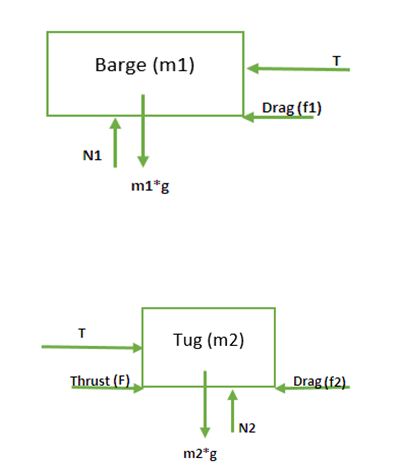Equations of Motion

Once the forces acting on the bodies are known, their equation of motion can be derived using Newton’s Second Law of Motion.
Some important assumptions and conventions followed throughout the report, unless explicitly mentioned, are given below:

• Throughout the report and associated Matlab codes, only SI units have been employed for all quantities.
• The (horizontal) position of the barge at time t with respect to an arbitrary origin is denoted by x1(t) and that of the tug is denoted by x2(t). Both the positions are in SI units (m).
• The tow cable is assumed to have a ‘natural length’ L = 100m. The value of the length is immaterial and can be replaced by any large enough number, but it is very helpful in the calculation of tension force in the tow cable. By large enough, it is implied that the cable should be long enough to avoid collision between the tug and the barge in case of a sag.
• The positive direction is taken as the forward direction i.e. the direction of motion of the tug and the barge

A list of various physical parameters of the system is given below to aid the application of Newton’s Second Law.

 Quantity/Variable Symbol Value Mass of Barge m1 2,300,000 kg Mass of Tug m2 110,000 kg Stiffness of tow cable k 3200 N/m Natural Length of tow cable L 100 m Barge Drag f1 92000*vbarge2 N Tug Drag f2 11000*vtug2 N Tug Thrust F Variable (Specified Later)

Barge’s Equation of Motion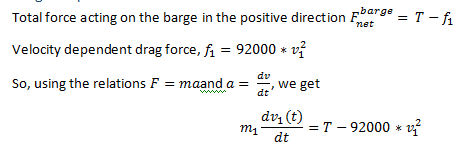Where v1(t) denotes the velocity of the barge at time t.

Tug’s Equation of Motion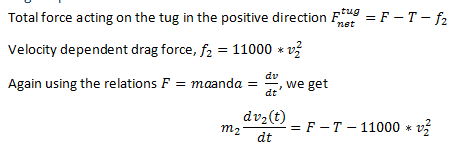Where v2(t) denotes the velocity of the tug at time t.

Thus, the equation of motion for the tug and the barge have been obtained. However, they can’t be solved in this form due to the presence of the term ‘T’. The tension in the tow cable depends on the elongation in the cable, which in turn depends on the position of the two boats. An explicit relation for tension in the tow cable is derived in the next section.

Tension in the Tow Cable
As mentioned earlier, the tension force in the tow cable is easily modeled if it is assumed that the cable has some ‘natural length’ at which it is fully taut but still has zero tension. Any further extension in its length will lead to tension due to its stiffness while any further decrease in its length will lead to sag. The numerical value of the natural length has no significance in the final answer, but is very helpful in the immediate steps.
There are three possible scenarios at any time t: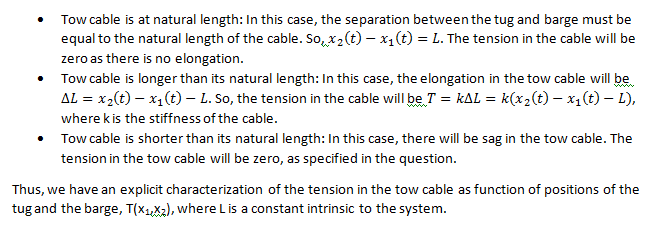#### Simulation of the Model

Converting into a System of Linear Differential Equations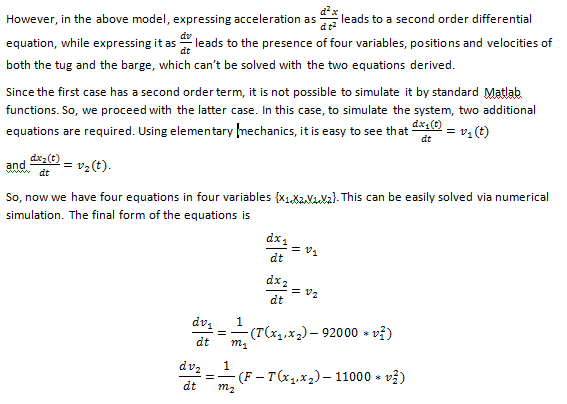This set of equations can be simulated in Matlab using ode23 and ode45 routines.

##### Simulation in Matlab

Thrust Function
In order to simulate the system of equations, we need to have an explicit function which outputs the thrust from tug for each time instant t. The thrust is specified as a piecewise linear function in the problem. Although Matlab doesn’t have a built-in piecewise linear function utility, the thrust pattern can be easily generated by using linear interpolation between the different ‘boundary’ points or ‘jerk’ points’, where the thrust changes abruptly.
The Matlab Code for the finding thrust using linear interpolation using interp1function and a plot of thrust over the time interval 0<t<1600 sec generated using this method is given below.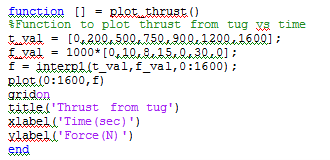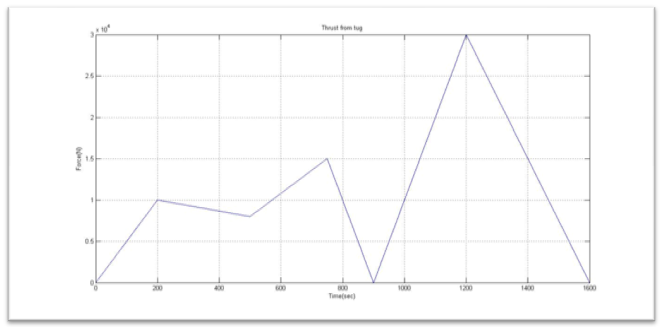The above plot exactly reproduces the pattern of thrust vs. time specified in the problem. So, this approach is working correctly.

Setting up the Differential Equation in Matlab
Matlab provides for a number of solvers to solve system of linear differential equations with initial conditions specified - ode45, ode23, ode113, ode15s, ode23s, ode23t, or ode23tb.
As specified in the problem, ode23 is used for carrying out numerical simulation. In order to be solved by Matlab, the equations should be in the format (dx_i)/dt=f(x_1,x_2,…,x_n,t) for all variables xi. The following code is used to set up the model in the desired form.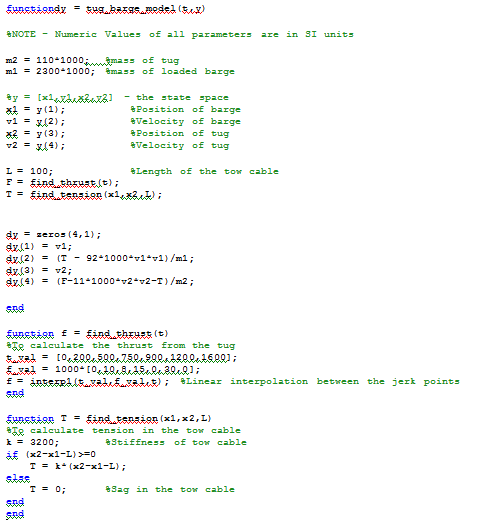The functions find_thrust and find_tension are used to find out the thrust from the tug and the tension in the tow cable at any instant.

Solving the Differential Equations
The system of equations is easily solved using the built-in ode23 solver in Matlab. The simulation is carried for 0<t<1600 sec. The initial positions of the barge and the tug are 0m and 100m respectively, so that the tow cable has zero tension but no slack. The initial velocities of both the boats are zero are stated in the problem statement. The data obtained is processed and plotted to be more meaningful for analysis and understanding. The code for simulation is given below:

####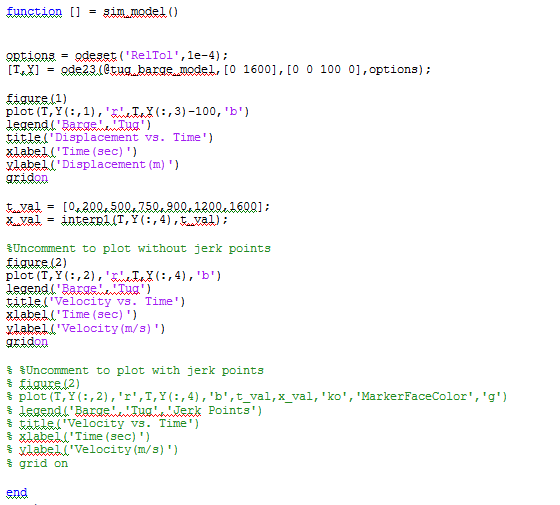Results

The plots generated by the function sim_model for the velocities of the two boats as well as their displacement from their initial positions are attached below: